Next: Klein Paradox for Spin-1/2 Up: Dirac Equation Previous: Combined PCT Symmetry

# Free-Particle Solutions and Wave Packets

Because of the completeness of plane-wave solutions, we may superimpose plane waves to construct localized wave packets. The wave packets are also solutions to the Dirac equation since it is linear.

A positive-energy wave packet solution can be written as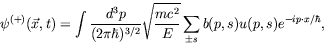(5.269)

where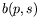is a complex scaler function. Normalized to unit probability gives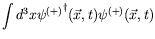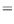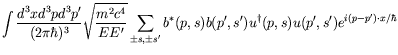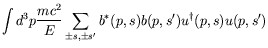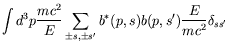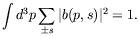(5.270)

The average current for such a wave packet is given by the expectation value of the velocity operator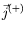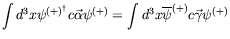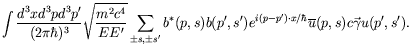(5.271)

The space part of the Gordon decomposition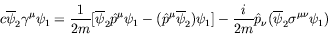(5.272)

can be used. The Gordon decomposition decomposes the Dirac current density into a convection current-density term, similar to the nonrelativistic case, and an additional spin current-density term. We can now write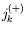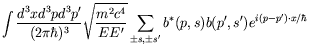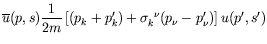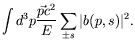(5.273)

Using the normalization condition, the current can be written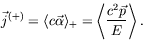(5.274)

Thus the average current for an arbitrary packet formed of positive energy solutions is just the classical group velocity. In the Schrödinger theory the velocity operator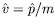is proportional to the momentum, but this is not the case in the Dirac theory. In the Dirac theory the velocity operator for a free particle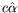is no longer a constant. We see that wave packets consisting of plane waves with only positive energy have the expectation value of the velocity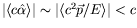, whereas the eigenvalues ofare exactly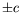. This motivates us to consider a wave packet containing both positive and negative energy solutions: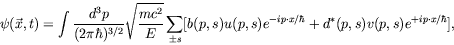(5.275)

whereand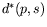are complex scalar functions. Normalized to unit probability, we have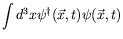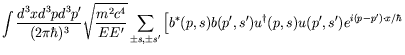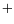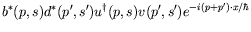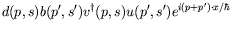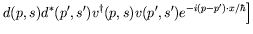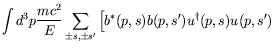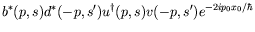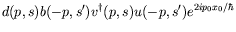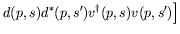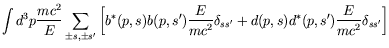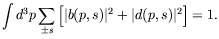(5.276)

A short calculation shows the current of the wave packet is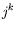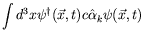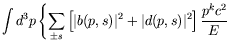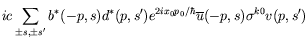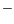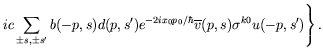(5.277)

The first term represents the time-independent group velocity that appeared before for positive energy only wave packets. The second and third terms are interferences of the solutions with positive and negative energy, which oscillate time-dependently because of the factors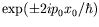. The frequency of this Zitterbewegung is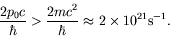(5.278)Next: Klein Paradox for Spin-1/2 Up: Dirac Equation Previous: Combined PCT Symmetry
Douglas M. Gingrich (gingrich@ ualberta.ca)
2004-03-18